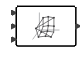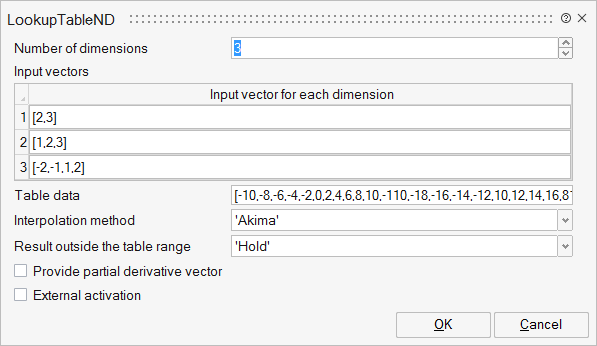# LookupTableND

This block approximates a non-linear function using interpolation on data provided as an "n-dimensional table".LookupTables

## Description

The LookupTableND block approximates a non-linear function using interpolation on data provided as a "ND-table". The N-dimensional table is then defined by specifying N vectors (X1, X2, ..., Xi, ..., Xn) of possibly different sizes (nXi), and a "ND-table", a vector of size (nX1*nX2*...*nXi*...*nXn). Output of the block is the approximation of the block input using the selected interpolation method.

Each input can be either scalar, vector or matrix.

## ParametersNameLabelDescriptionData TypeValid Values

nDims

Number of dimensions

Number of dimensions of the lookup table. One dimension corresponds to one input.

Number

dims

Input vectors

Structure

dims/dimi

Input vector for each dimension

A vector indicating input break points for each dimension.

Cell of vectors

ff

Table data

A vector containing data for n-dimensional table.

Vector

Method

Interpolation method

String

'Hold'
'Nearest'
'Linear'
'Akima'
'Fritsch_Butland'
'Steffen'

DoOutside

Result outside the table range

String

'Hold'
'Linear'
'Zero'
'Error'

derivative

Provide partial derivative vector

Select for the block to provide the vector of the partial derivative of the table with respect to each dimension.

Number

0
1

externalActivation

External activation

Specifies whether the block receives an external activation or inherits its activation through its regular input ports. When External Activation is selected, an additional activation port is added to the block. By default, external activation is not selected.

Number

0
1

## Ports

NameTypeDescriptionIO TypeNumber

Port 1

explicit

output

1

Port 2

explicit

output

derivative

Port 3

explicit

input

nDims

Port 4

activation

input

externalActivation